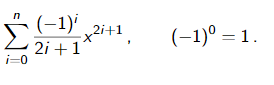# Sum of a Series, Help!

13 views (last 30 days)
Nathan Barnes on 9 Jul 2020
Commented: Rena Berman on 22 Jul 2020 at 18:26
Hi i have had a look around for some help on multiple variables using sum and sigma.
Couldnt quite understand how to apply those examples to my problem.
And I have got to write out this function and be able to plot it on a graph.
Being that n is 1,2 or 3 (on the same graph)
Pn(x) =Stephen Cobeldick on 10 Jul 2020
"Sum of a Series, Help!"
Hi i have had a look around for some help on multiple variables using sum and sigma.
Couldnt quite understand how to apply those examples to my problem.
And I have got to write out this function and be able to plot it on a graph.
Being that n is 1,2 or 3 (on the same graph)madhan ravi on 10 Jul 2020
Thank you Stephen!
Rena Berman on 22 Jul 2020 at 18:26

David Hill on 9 Jul 2020
Not sure exactly what you are asking.
x=0:.1:10;
for n=1:3
S(n,:)=arrayfun(@(i)sum(repmat(-1,1,n+1).^(0:n)./(2*(0:n)+1).*repmat(i,1,n+1).^(2*(0:n)+1)),x);
end
subplot(3,1,1);
plot(x,S(1,:));
subplot(3,1,2);
plot(x,S(2,:));
subplot(3,1,3);
plot(x,S(3,:));

madhan ravi on 9 Jul 2020
See after providing a complete solution usually the OP deletes the question. That’s why it’s better to provide hints than a complete solution.
Nathan Barnes on 9 Jul 2020
It is correct and I appreciate it very much, I'm sorry if I came off as ungreatful.
Rik on 9 Jul 2020
Then why did you delete it?

Boxn Hen on 9 Jul 2020
Hope to help you as follow.Ps:I just use 'for' to sum all seperate results.
function result=SumAndSigma(x,n)
r(1)=0;
for i=0:n
r(i+2)=r(i+1)+((-1)^i/(2*i+1)).*x.^(2*i+1)% There are n+1 elements and the first one is 0.
end
result=r(2:end);
end
And input x and n to the function SumAndSIgma.
%for example:x=3;n=5
x=3;n=5;
result=SumAndSigma(x,n)
data=0:n;
plot(data,result);